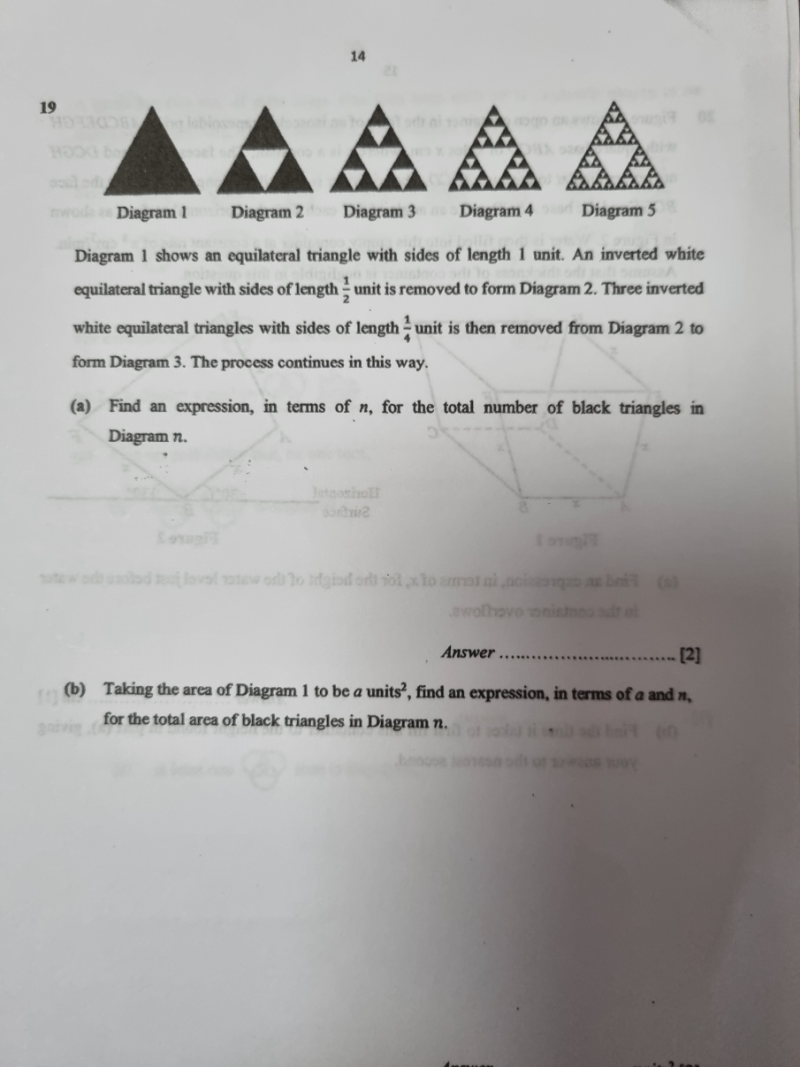# QuestionThank you

(a)  Diagram       No. of black triangles

1                           1     =    30

2                          3     =    31

3                          9     =    32

4                         27    =    33

5                         81    =    34

n                          ?      =    3n-1

Hence number of black triangles for the nth diagram = 3n-1

(b)  Diagram           Area of Diagram(black triangles)

1                           a                             =  (30 / 20 ) a

2                          (3/4) a                  =  (31 / 22 ) a

3                          (9/16) a                =  (32 / 24 ) a

4                          (27/64) a              =  (33 / 26 ) a

5                           (81/256)a            =  (34 / 28 ) a

n                                ?                       = (3n-1 / 22(n-1) ) a  = a (3/4)(n-1)

Hence the area of black triangles in Diagram n is   a(3 / 4) (n-1)

0 Replies 1 Like ✔Accepted Answer